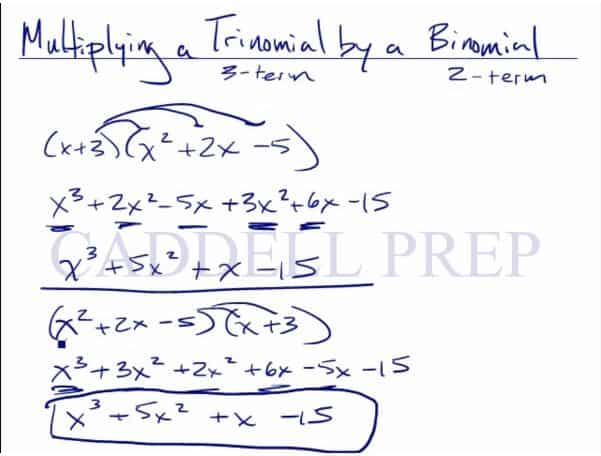In this video, we are going to look at how to multiply a trinomial by a binomial. After you finish this lesson, view all of our Algebra 1 lessons and practice problems.

For example:
To multiply$(x+3)(x^2+2x-5)$ we have to distribute each term in the binomial to each term in the trinomial.
When we distribute the x to the terms in the trinomial, we get$x^3$,$2x^2$, and$-5x$. When we distribute the 3 to the trinomial, we get$3x^2$,$6x$, and$-15$. So now we are left with$x^3+2x^2-5x+3x^2+6x-15$
From here, we want to combine like terms, to give us a final answer of$x^3+5x^2+x-15$Examples of Multiplying A Trinomial By A Binomial

Example 1$Solve (x + 3)(x^2 - 5x + 7)$

Distribute each term into the second expression$x^3-5x^2+7x+3x^2-15x+21$

Then combine like terms$x^3-2x^2-8x+21$

Example 2$Solve (2a + b)(2a^2 - 5ab + 3b^2)$

Distribute each term into the second expression$2a^3-10a^2b+6ab^3+2a^2b-5ab^2+3b^4$

Then combine like terms$2a^3-8a^2b+6ab^3-5ab^2+3b^4$

Video-Lesson Transcript

Let’s go over how to multiply a trinomial by a binomial.

Trinomial has three terms and a binomial has two terms.

Let’s go over multiplying a binomial by a binomial first.

We will just distribute each term into the second expression then combine like terms.

For example:$(x + 3)(x - 4)$$x^2 - 4x + 3x - 12$$x^2 - 1x - 12$

Now, let’s try a binomial times a trinomial.$(x + 3)(x^2 + 2x - 5)$

So, let’s distribute each term into each term of the second expression.$x^3 + 2x^2 - 5x + 3x^2 + 6x - 15$

Then combine the like terms$x^3 + 5x^2 + x - 15$

And we can also do the same thing the other way around.$(x^2 + 2x - 5)(x + 3)$

Here, we distribute each term of the trinomial into each term of the binomial.$x^3 + 3x^2 + 2x^2 + 6x - 5x -15$

It may look different. But if you look closely, we have the same exact terms whereas you did up here, just in different orders.

So now, when we combine like terms, we’ll have the same result.$x^3 + 5x^2 + x - 15$

As you can see, we have the same answer.

It doesn’t matter which way you distribute. Just make sure you distribute both of them.